Next: Probabilistic Time Series Modeling Up: Temporal Modeling Previous: Principal Components Analysis

# Pre-Processing Implementation and Exponential Memory Decay

It should be noted that the above analysis actually used weighted versions of the Y vectors to include a soft memory decay process. Figure 4.4 depicts the pre-processing, exponential weighting and dimensionality reduction. Instead of abruptly cutting off the Y at the T'th sample of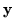, a smooth decay is applied to more distant observations.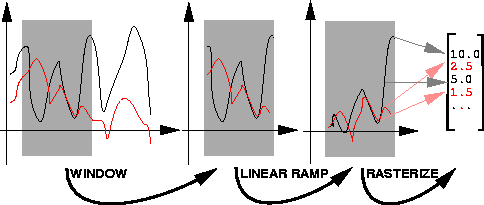Recall that the large vectors Y(t) represent a full window of past interaction (short term memory). This window effectively covers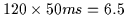seconds of temporal data. This data is weighted with an exponential decay which scales downvectors that constitute the big Y(t) vector. The further back in time acomponent is, the more its amplitude is attenuated. Thus, an exponential ramp function is multiplied with each Y window (i.e. a few seconds of each of the 30 time series). This reflects our intuition that the more temporally distant the elements in the time series, the less relevant they are for prediction. This decay agrees with some aspects of cognitive models obtained from psychological studies . Once the vectors have been attenuated, they form a new 'exponentially decayed' short term memory window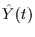. The process is shown in Figure 4.4 where a window is placed over the time series, generating a short term memory Y. An exponential decay function is used to decay it and generates the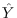version. The eigenspace previously discussed is really formed over thedistribution and representingin only this subspace (i.e. the top eigenvectors) generates the compact vector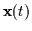. This is the final, low dimensional representation of the gestural interaction between the two humans over the past few seconds.Next: Probabilistic Time Series Modeling Up: Temporal Modeling Previous: Principal Components Analysis
Tony Jebara
1999-09-15